# Find word with highest number of repeated letters in string

Problem Statement: Write a program to find a word in a given string that has the highest number of repeated letters. If not found, return -1.

Examples:

```Example 1:
Input: string=”abcdefghij google microsoft”
Explanation: In “google” g appears 2 times, o appears 2 times which is highest among all words

Example 2:
Input: string = “cameron blue”
Output: -1
Explanation: No word has more than 1 letter.
```

### Solution:

Disclaimer: Don’t jump directly to the solution, try it out yourself first.

### Intuition:

• For a word, we will calculate the frequency of each letter.
• If any letter appears more than 1, then that word can be the solution. The frequency of letters for each word will be calculated separately .
• The above process is done with all the words present in the string
• At the end the word with the highest number of such repeated letters will be our answer.

### Approach:

• We will be using 2 pointers left and right namely.
• Left will be starting from index 0 and the right will be starting from the index which is next to the left.
• Now , till we get “ “ or end of the string we will increment the right pointer. Whenever we get “ “ , this means we covered 1 word.
• Now it’s time to check the frequency of each letter of the current word between the left and right pointer. For this we will be hashing each letter in the frequency array.
• If any letter appears more than 1, then the current word can be the answer.
• This process goes on till we check this for each word of the string.
• Finally we will print the word with the highest number of repeated letters.

### Dry Run: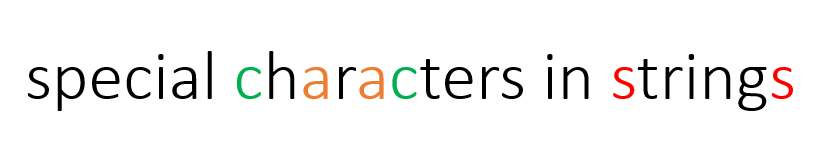Left pointer = red pointer , Right pointer = blue pointer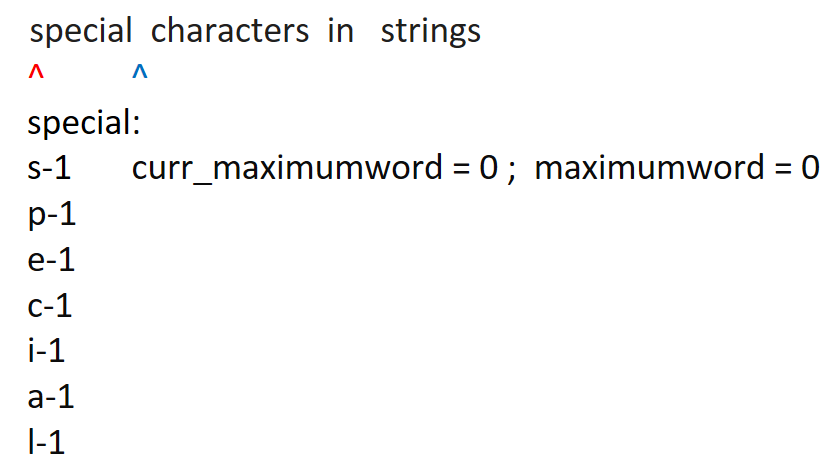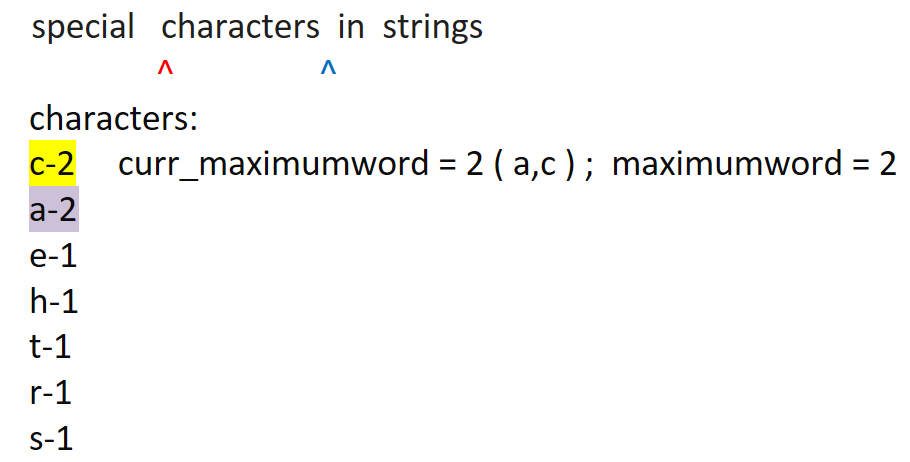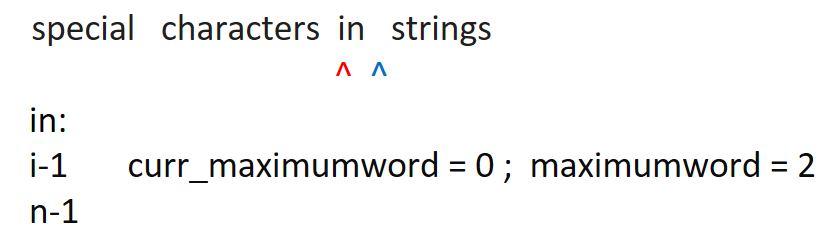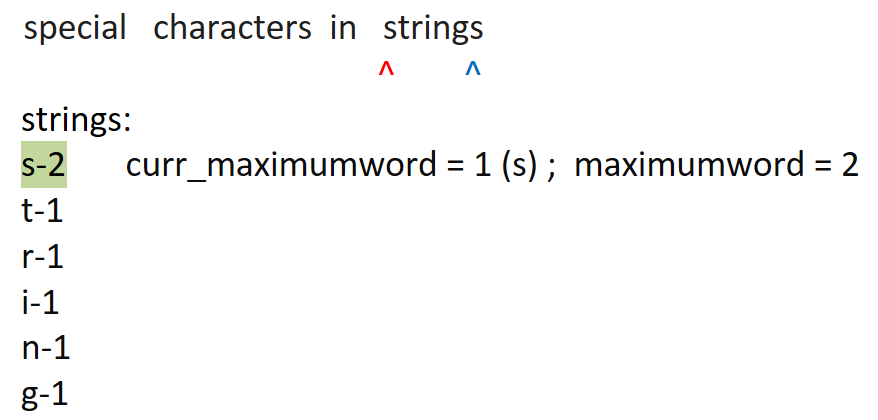Code:

## C++ Code

``````#include <bits/stdc++.h>
using namespace std ;

class Solution {
public:
void HighestRepeatedLetters(string &str) {
int len = str.length() ;

int maximumword = 0 ;

int curr_maximumword = 0  ;

string result = ""  ;

for (int left = 0; left < len;) {

int right = left + 1 ;
while (str[right] != ' ' && right < len) {
right++ ;
}

int frequency = {0}  ;
curr_maximumword = 0  ;

for (int index = left ; index < right ; index++) {
frequency[str[index] - 'a']++  ;
}
for (int index = 0 ; index < 26 ; index++) {
if (frequency[index] > 1) {
curr_maximumword ++  ;
}
}

if (curr_maximumword > maximumword) {
maximumword = curr_maximumword ;
result = ""  ;
for (int j = left ; j < right ; j++)
result += str[j] ;

}

left = right + 1  ;
}

if (result.empty()) {
cout << "-1";
}
else {

cout << "Word with highest number of repeated letters : " ;
cout << result << "\n"  ;
}
}
};
int main() {

string str = "abcdefg google microsoft"  ;
Solution obj ;
obj.HighestRepeatedLetters(str)  ;
return 0 ;
}
``````

Output:

Word with highest number of repeated letters : google

Time complexity: O(n^2)

Reason: Outer Loop is used to traverse the Left pointer. Updating frequency array, traversing right pointer, updating curr_maximumword are part of the inner loop.

Space complexity: O(1)

## Java Code

``````import java.util.*;

class Solution {
static void HighestRepeatedLetters(String str) {
int len = str.length() ;

int maximumword = 0 ;

int curr_maximumword = 0  ;

String result = ""  ;

for (int left = 0; left < len;) {

int right = left + 1 ;
while (right < len && str.charAt(right) != ' ') {
right++ ;
}

int frequency[]=new int  ;
curr_maximumword = 0  ;

for (int index = left ; index < right ; index++) {
frequency[str.charAt(index) - 'a']++  ;
}
for (int index = 0 ; index < 26 ; index++) {
if (frequency[index] > 1) {
curr_maximumword ++  ;
}
}

if (curr_maximumword > maximumword) {
maximumword = curr_maximumword ;
result = ""  ;
for (int j = left ; j < right ; j++)
result += str.charAt(j) ;

}

left = right + 1  ;
}

if (result.equals("")) {
System.out.println("-1");
}
else {

System.out.print("Word with highest number of repeated letters : ") ;
System.out.println(result)  ;
}
}

public static void main(String args[]) {

String str = "abcdefg google microsoft"  ;
HighestRepeatedLetters(str)  ;

}
}``````

Output:

Word with highest number of repeated letters : google

Time complexity: O(n^2)

Reason: Outer Loop is used to traverse the Left pointer. Updating frequency array, traversing right pointer, updating curr_maximumword are part of the inner loop.

Space complexity: O(1)

Special thanks to Shreyas Vishwakarma for contributing to this article on takeUforward. If you also wish to share your knowledge with the takeUforward fam, please check out this article